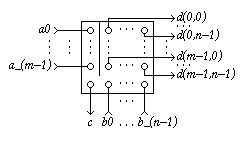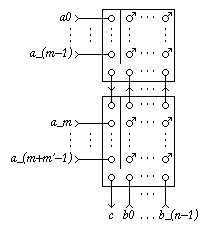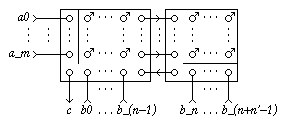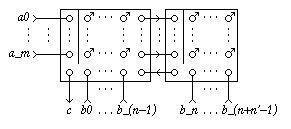EDIS: Guide | FAQ | New | Search | Bibliography | Index | Feedback

# mxn-Decision Call

## Specifications

### Informal

An mxn-Decision Call (m>0, n>0) has m+n input terminals a_i (0<=i<m) and b_j (0<=j<n), and mn+1 output terminals c and d_i_j. A signal appearing on either of the a_i terminals will cause a signal to be produced at terminal c. The combination of a signal at terminal a_i and terminal b_j will cause a signal to be produced at terminal d_i_j. The environment must guarantee mutual exclusion of the a_i-inputs and also of the b_j inputs.

### XDI

Schematic diagram for a mxn-Decision Call:[Zoom|FIG]

### Verdect

Parameterized definitions are not possible in VERDECT, but this sketch gives the general idea:

Specification in Verdect:

```
define DCALL[m,n]( a0?, ..., a(m-1)?, b0?, ..., b(n-1)?,
c!, c(0,0)!, ..., c(m-1,n-1)! ) =
pref *[ (a0? | ... | a(m-1)?); c! ]
|| pref *[ (a0? || b0?); d0!
| ...
| (a(i)? || b(j)?; d(i,j)!
| ...
| (a(m-1)? || b(n-1)?; d(m-1,n-1)!
]
end
```

### DI Algebra

The specification of the case m,n=3 is as follows:

Specification in DI Algebra.

. (Not available for general m and n.)

## Properties

XDI Report.

The roles of subscripts i can be permuted and, independently also the roles of subscripts j can be permuted, provided that the d_i_j outputs are permuted accordingly.

The mxn-Decision Call satisfies Rules Y' and Z^out, but not Z^inp (choice between a_i inputs, and also between b_j inputs).

## Implementations

### DI Decompositions

1. An mxn-Decision Call with m=k and n=1 is a k-Call.
2. An mxn-Decision Call with m,n=2 is a Decision Call.
3. An mxn-Decision Call can be implemented with m Forks, a k-Merge with k=m, and an mxn-Decision-Wait (equ):[Zoom|FIG]
4. An mxn-Decision Call with m=k+k' can be implemented with an mxn-Decision Call with m=k and an mxn-Decision Call with m=k'+1 (equ):[Zoom|FIG]
5. An mxn-Decision Call with n=k+k' can be implemented with an mxn-Decision Call with n=k+1 and an mxn-Decision Call with n=k' (equ):[Zoom|FIG]
Note the orientation of the an mxn-Decision Call with m,n=n',m on the right. Using an mxn-Decision Call with m,n=m,n' instead does not work (deadlock): INCORRECT[Zoom|FIG]
6. Observe that a Decision Call can be implemented with one Latch one Merge, and two Forks. By suitably combining this implementation with scheme 4 and 5 above, one can obtain implementations of mxn-Decision Calls, for arbitrary m,n>1, in terms of (m-1)(n-1) Decision Calls, (m-1)(n-1) Merges, and 2(m-1)(n-1) Forks.

The resulting implementation does depend on how schemes 4 and 5 are applied. This is readily verified by considering the two decompositions of an mxn-Decision Call with m,n=3' into four Decision Calls.

### Using Boolean Gates

No information available

### Using Transistors

No information available

## Generalizations

No information available

[Dickson74]

[Dickson74]